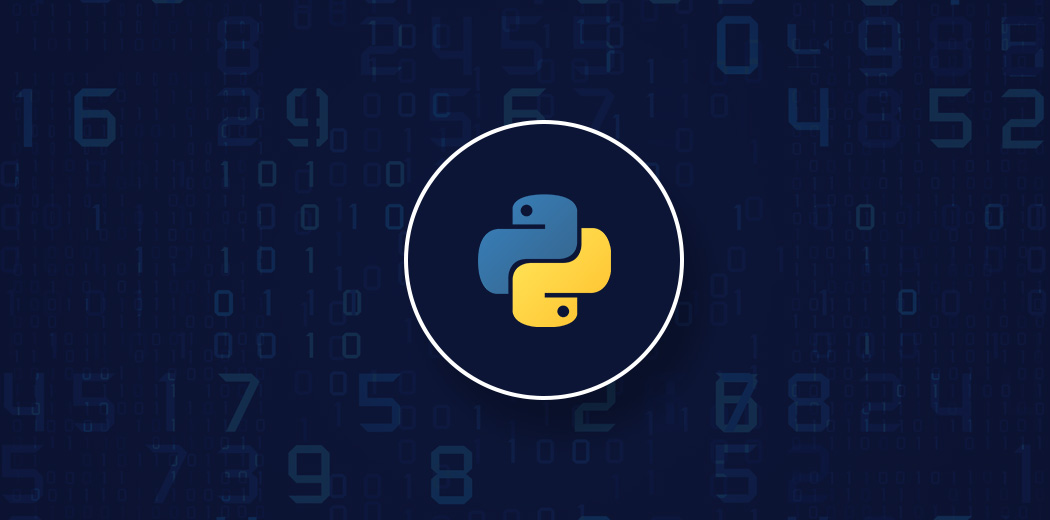guest_blog — Published On September 5, 2020 and Last Modified On September 2nd, 2022

## Introduction• Enables automatic and explicit data alignment.
• Allows intuitive getting and setting of subsets of the data set.

```# for getting values with a boolean array
print (df.loc['a']>0)```
`print df.loc[:,'B']`

## The query() Method

```#creating dataframe of 10 rows and 3 columns
df4 = pd.DataFrame(np.random.rand(10, 3), columns=list('abc'))
df4```
```#with query()
df4.query('(x < b) & (b < c)')```
• drop_duplicates: removes duplicate rows.
```df5 = pd.DataFrame({'a': ['one', 'one', 'two', 'two', 'two'],
'b': ['x', 'y', 'x', 'y', 'x'],
'c': np.random.randn(5)})
df5```
`df5.duplicated('a')`
`df5.drop_duplicates('a')`